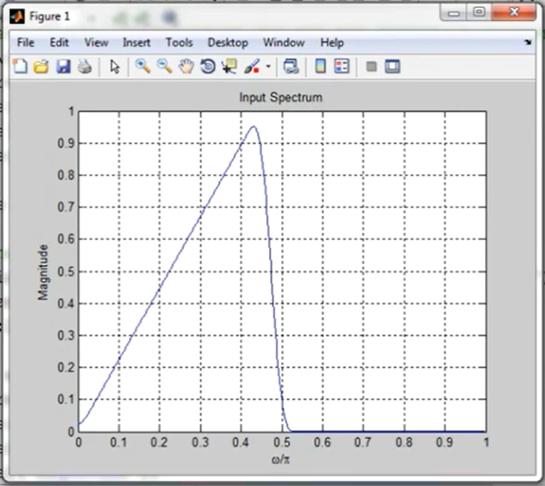Open in App
Not now

# Upsampling in Frequency Domain in MATLAB

• Difficulty Level : Medium
• Last Updated : 04 Jan, 2023

Upsampling” is the process of inserting zero-valued samples between original samples to increase the sampling rate. (This is called “zero-stuffing”.) Upsampling adds to the original signal undesired spectral images which are centered on multiples of the original sampling rate.

In Frequency domain , Upsampling means the padding of zeros at the last of max frequency components on all sides of the signal.

Now we see the upsampling process in the frequency domain. Here we understand Upsampling in frequency domain by one example of MATLAB program :

## Matlab

 % MATALB program to illustrate the% upsampling process in the frequency domain.% Program for effect of upsampling in the frequency domain% Bandlimited signal to be interpolated freq = [0 0.45 0.5 1];mag = [0 1 0 0];x = fir2(99, freq, mag); % Evaluate the interpolation and% see the effect in frequency domain[Xz, w] = freqz(x, 1, 512);figure, plot(w/pi, abs(Xz)); gridxlabel('\omega/\pi');ylabel('Magnitude');title('Input Spectrum'); pause % Generation of interpolated signalL = input('Enter the integer factor for Upsampling = ');y = zeros(1, L*length(x));y([1:L:length(y)]) = x; [Yz, w] = freqz(y, 1, 512);figure, plot(w/pi, abs(Yz)); gridtitle ('Output Spectrum');xlabel('\omega/\pi');ylabel('Magnitude');

Output:### Code Explanation :

To visualize the effect of the frequency domain first then we require the input signal to be operated here, basically to the input signal which is operated here should be a Bandlimited signal. here we have a Bandlimited and input signal to be interpolated so for a frequency domain we require to define one variable as freq to generate frequency we take example freq = [0 0.45 0.5 1] and for another excess, we require the magnitude as well and then we defined one variable x and we need to take syntax fir2 to the utilization and within the parenthesis, we provide 99, freq,  mag variables name which we defined then the evaluation for upsampling that is the upsampling process also the visualization corresponding signal into the frequency domain for this purpose we defined two variable together Xz and w this can opt from MATLAB  syntax freqz we providing input arguments are x,1,512 that is the very important syntax to get the details of syntax freqz we can know more about it by writing

help freqz

in the command window.  freqz is the Digital filter frequency response generation of the frequency domain. and now for see the effect we have to plot it in separate MATLAB window we use figure as a keyword and then use plot command  and for plot command we require two input arguments corresponding to input we take first input w/pi and second input  abs and then mentioning grid  and then we use xlabel command for nomenclature we   use omega and pi these are two constant representation here we take another ylabel in which we take Magnitude  then we take title input spectrum and then for see the effect of the interpolation we use keyword pause and then we generating upsampling signal we again take two variable L and y to defined  all done process we use input command which is printed in command window when we execute the program, and we accepting integer factor for interpolationthen we defining one variable zeros and in this we provide length of  x which we defined in above steps in code then we getting two variable yz and w and command freqz and by figure command we plot it and again take two input w/pi and abs and then we provide title Output spectrum and along with the title for horizontal axis we defined xlabel and another axis ylabel Magnitude .

that is the complete MATLAB script for the Upsampling in Frequency Domain MATLAB program, first of all, we need to save it in our directory, and the output (effect of upsampling in frequency domain ) is shown in above image .

My Personal Notes arrow_drop_up